# 直方图均衡化和空间滤波

## 1习题

### 1.1 直方图均衡化

L为图像灰度级， Pr(rj) $P_r(r_j)$表示原始灰度值 rj $r_j$对应出现的概率，直方图均衡化公式：

sk=T(rk)=(L1)j=0kPr(rj)

=(L1)MNj=0knj

k=0,1,2...,L1

rj $r_j$对应的像素灰度经过直方图均衡化后变为 sk $s_k$，所以变的是对应的灰度级，而对应灰度级的累积分布概率积分CDF不变，让 Ps(rj) $P_s(r_j)$表示直方图均衡化后灰度值 rj $r_j$对应出现的概率,则有；

j=0kPr(rj)=j=0kPs(rj)

k=0,1,2,,,L1

### 1.2 空间滤波

85169181213815534202431635080204480

101101101

1. 用给定的滤波器对这张灰度图（边界补零）进行卷积，写出卷积后的结果（大小应为 4 x
4）。

17775131173420218324336271249107362263107133207

1. 请说出你得到的卷积结果中正数和负数分别表示什么含义。

1. 根据你所学到的知识， 谈一谈题目中给出的 3 x 3 滤波器可以有哪些应用。

## 2编程题

### 2.1直方图均衡化

1. 计算并显示图像的直方图， 并把结果粘贴到报告里。注意：你必须用你自己实现的函数
来计算直方图，但是允许调用现成的 API 来显示直方图。 (例如，你不能调用 Matlab 的“imhist”
来计算直方图，但是可以调用“subplot” , “hist”来显示直方图。 )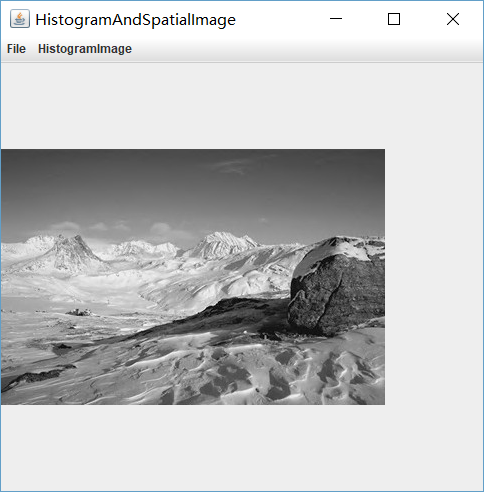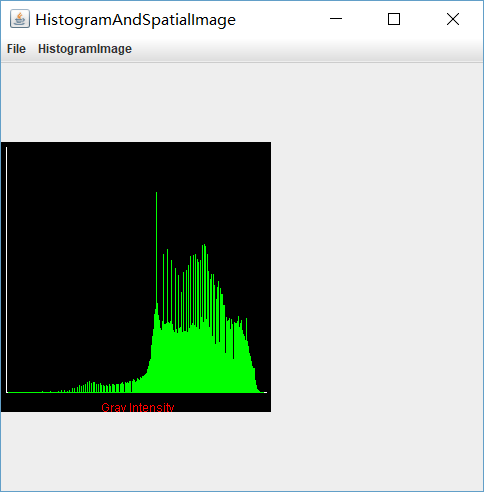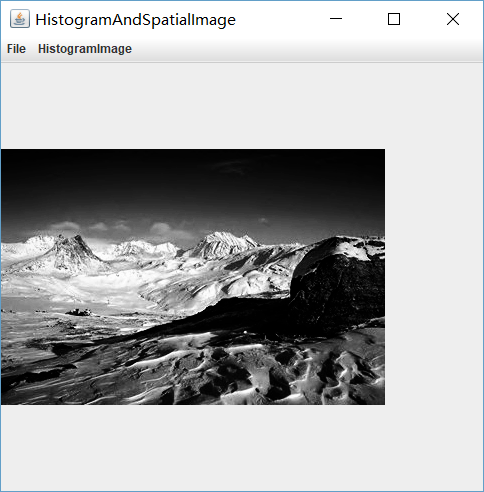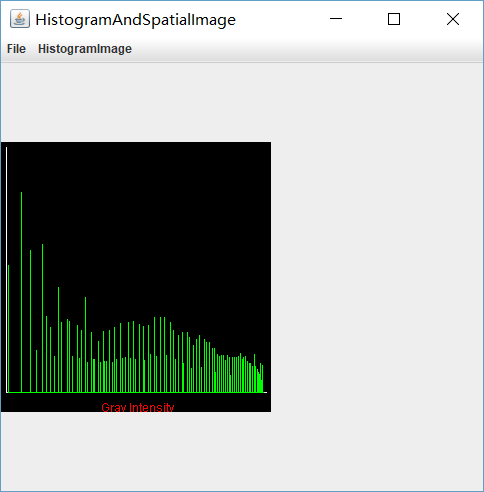1. 分析直方图均衡化后的结果，字数不能超过一页。

3.详细描述你是如何实现直方图均衡化操作的，也就是说，针对“equalize_hist”函数进

(L1)MNj=0knj

    double k = (double)255 / (double)(height * width);
double[] c = new double;
c = k * array;
for (int i = 1; i < 256; i++) {
c[i] = c[i - 1] + k * array[i];
}

### 2.2空间滤波

1. 分别用 3 x 3， 7 x 7 和 11 x 11 的均值滤波器来平滑你输入的图像，将相应的三个输出结
果粘贴到报告里。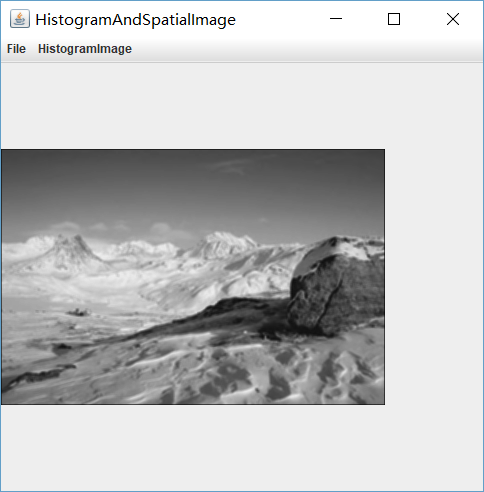3×3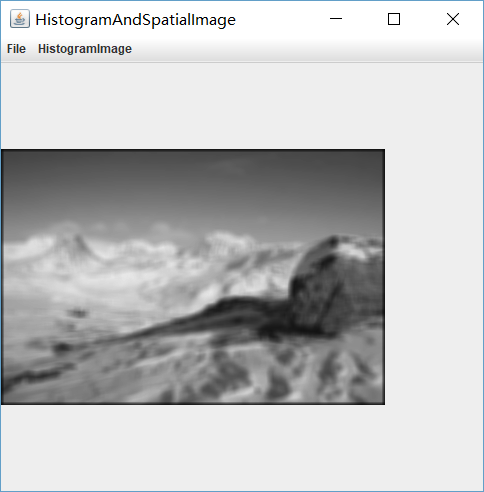7×7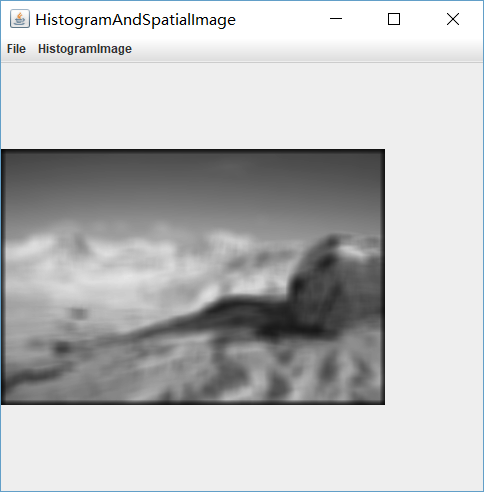11×11

2.用 3 x 3 的拉普拉斯滤波器来锐化你输入的图像（课本上有 4 种拉普拉斯滤波器，参见图
3.37， 你可以使用其中任意一种），并将输出结果放在报告中。除此之外，请简单介绍一下为什

0101410103.将高提升滤波（high-boost filter） 用在输入的图像中 （也就是说， g(x,y)=f(x,y)+kgmax(x,y) $g(x,y)=f(x,y)+k*g_max(x,y)$其他细节参见课本式(3.6-9)）。过程中涉及的平滑部分应用用课本图 3.32(a)所示的滤波器来完成。请自行选择合适的 k（式(3.6-9)中的权值）。在报告中，你需要说明你选择的 k的值，并贴上对应的输出结果。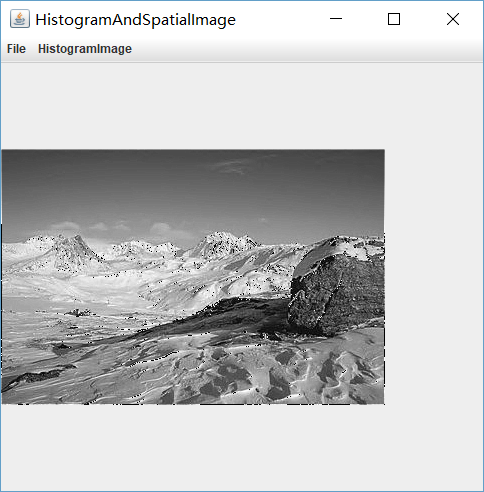1. 详细描述你是如何实现空间滤波操作的，也就是说，针对“filter2d”函数进行算法说
明，字数不能超过两页。

g(x,y)=s=aat=bbw(s,t)w(s,t)f(x+s,y+t)

public int[][] getFilteringArray(int[][] imageArray, int[][] filteringArray) {
int height = imageArray.length, width = imageArray.length;
int[][] temp = new int[height][width];
for (int i = 0; i < height; i++) {
for (int j = 0; j < width; j++) {
temp[i][j] = getFilteringValue(i, j, imageArray, filteringArray);
}
}
return temp;
}

public int getFilteringValue(int row, int col, int[][] imageArray, int[][] filteringArray) {
int count = 0;
int height = imageArray.length, width = imageArray.length;
int filterLength = filteringArray.length;
int gap = (filterLength - 1) / 2;
for (int i = 0; i < filterLength; i++) {
for (int j = 0; j < filterLength; j++) {
if (row + i - gap >= 0 && row + i - gap < height &&
col + j - gap >= 0 && col + j - gap < width) {
count += imageArray[row + i - gap][col + j - gap];
}
}
}
return count / (filterLength * filterLength);
}10-201万+
09-011万+
11-05856
01-202万+
06-22188
11-291万+
11-295万+
03-271525
08-141万+
11-02
04-089464
10-201554
11-196861
07-11147
05-013574
11-102911
10-14567
04-011575